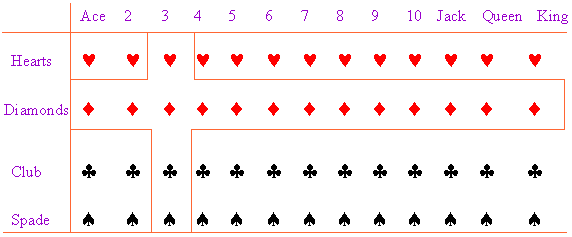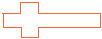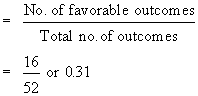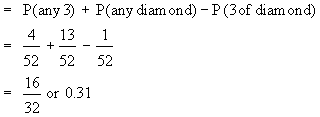Support the Monkey! Tell All your Friends and TeachersHome MonkeyNotes Printable Notes Digital Library Study Guides Study Smart Parents Tips College Planning Test Prep Fun Zone Help / FAQ How to Cite Request a New Title

7.5 The  Laws  of  Probability

So  far we have discussed probabilities of single events.  In many situations we come across two or more events occurring together.  If  event  A and  event B  are two events and either A or B or both occurs, is denoted by  A  Ç  B  or  (A + B) and the event that both A and B occurs is denoted by  A Ç B or  AB.  We term these situations  as compound event or the joint occurrence of events.  We  may  need  probability  that  A  or  B  will  happen.

It is denoted by  P (A Ç  B)  or  P (A + B).  Also we may need the probability that A and B (both) will  happen simultaneously.  It is denoted by  P (A Ç  B) or  P (AB).

Consider a situation, you are asked to choose any 3 or any diamond or both from a well shuffled pack of 52 cards.  Now you are interested in the probability of this situation.

Now see the following diagram.

It is denoted by P (A È B) or P (A + B). Also we may need the probability that A and B (both) will happen simultaneously. It is denoted by P (A Ç B) or P (AB).

Consider a situation, you are asked to choose any 3 or any diamond or both from a well shuffled pack of 52 cards. Now you are interested in the probability of this situation.

Now see the following diagram.Now count the dots in the areawhich fulfills the condition any 3 or any diamond or both. They are 16.

Thus the required probabilityYour browser does not support the IFRAME tag.

In the language of set theory, the set any 3 or any diamond or both is the union of the sets any 3 which contains 4 cards  and any diamond which contains 15 cards. The number of cards in their union is equal to the sum of these numbers minus the number of cards in the space where they overlap. Any points in this space, called the intersection of the two sets, is counted here twice (double counting), once in each set. Dividing by 52 we get the required probability.

Thus P (any 3 or any diamond or both)In general, if the letters A and B stands for any two events, then

Index

7. 1 Introduction
7.2 Trial
7.3 Sample Space
7. 4 Definition of Probability
7. 5 The Laws of Probability
7. 6 Conditional Probability
7. 7 Theoretical Distribution
7. 8 Binomial Distribution
7. 9 Normal Distribution
Chapter 8Search: All Products Books Popular Music Classical Music Video DVD Toys & Games Electronics Software Tools & Hardware Outdoor Living Kitchen & Housewares Camera & Photo Cell Phones Keywords: